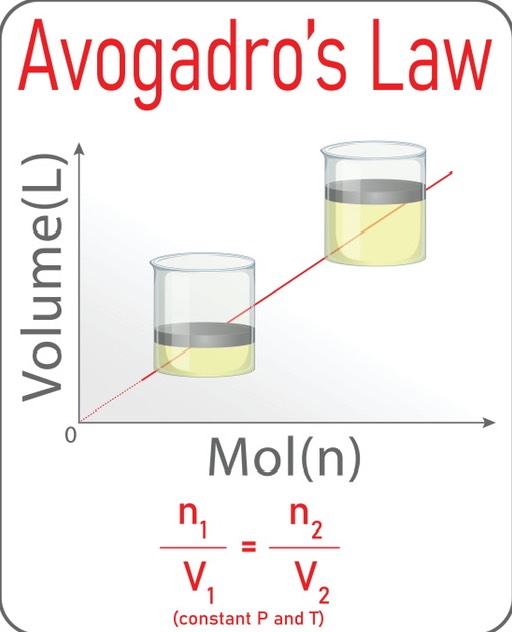×## Introduction

For giving an idea about the relationship between gas pressure, the volume of gas, the number of moles of gas, and temperature, gas laws are crucial. The law establishes a correlation between volume and molecule count, just like other Avogadro rules for gases. He noticed that there is a direct relationship between the gas volume and the number of gas molecules. According to this law, all gases contain an identical number of molecules in an equal volume when the temperature and the pressure of the gas are also identical. So it is clear, under the same circumstances, the number of gas particles in all equivalent gases is constant.

## Derivation of the formula of Avogadro’s law

This law states: “equal volumes of all gases, at the same temperature and pressure, have the same number of molecules”. According to Avogadro's law, the number of gas particles(n) and volume of the gas(V) are directly proportional whereas the pressure(P) and the temperature of the gas(T) are constant. So the formula is V ∝ n.

It can be derived in the following way.

Ideal gas equation is PV = nRT……1)

So,Now, the pressure of the gas P and temperature T are constant. And R is the universal gas constant.

So,Then V ∝ n ……2)

This equation 2) is known as Avogadro’s law.

Considering, the volume of the gas changes from V1 to V2 and the number of gas particles becomes n2 from n1.

As we know,So,This equation 3) is another form of Avogadro’s law.

## Graphical representation of Avogadro’s law:

Avogadro’s law is V ∝ n

then V = Kn (K is constant)

If we plot volume V vs. number of gas particles n, we get a straight line and the slope of this line is K.1. Determination of the amount of gas:

At STP conditions, the molar volume of the gas can be measured by Avogadro’s law. Under this condition, the molar volume of the gas is always constant, that is 22.4 litre or 22400 ml. Applying this value, an unknown amount of gas molecules are calculated.

1. Helps in breathing:

Because of the intake of air throughout respiration, the lungs expand. When we breathe, the number of particles of gases increases, increasing the volume of the lungs.

1. Balloon inflation:

The introduction of air causes balloons to extend. The balloon’s volume will

expand as the volume of air does.

1. It is suitable for only ideal gases. Real gases are applicable only when pressure decreases and the volume of the gas increases.

2. This law is not valid for gases like hydrogen, and helium, which have a low molar mass.

3. It is applicable for a system with very low concentration where there is no interaction between the gas molecules.

## Summary:

One of several major gas laws that deal with multiple moles and volumes of gas is Avogadro's law. All gases of equal volume have the same number of gas particles when they remain at the same temperature and pressure. A straight line is generated when we plot the volume of the gas V with the number of gas particles n in the graph. This law(V1n2=V2n1) has various applications in calculating unknown amounts of gas, breathing and balloon inflation, and many others. But it has some limitations in the case of real gases and gases having low molecular weights.

1. Why doesn't Avogadro's law apply to solids and liquids?

Ans: Gases are substantially different from solids and liquids. The intermolecular attraction in solids and liquids is much higher than in gases. Due to this interaction, solids and liquids have unique shapes and features. So this law does not apply to solids and liquids.

2. How is the law of Avogadro applied in real life?

Ans: The additional air volume assists the flat tyre to restore its former shape as soon as the necessary amount of air has been supplied. As a result, the expansion of flat tyres serves as a practical illustration of Avogadro's law.

3. Why do gases have the same amount of space?

Ans: Based on  Avogadro's hypothesis, an identical number of particles are contained by the gases having the same volume at a specific pressure and temperature. The dimensions of the particles are small enough, as the majority of a gas's volume is composed of void spaces.

#### Recent Posts